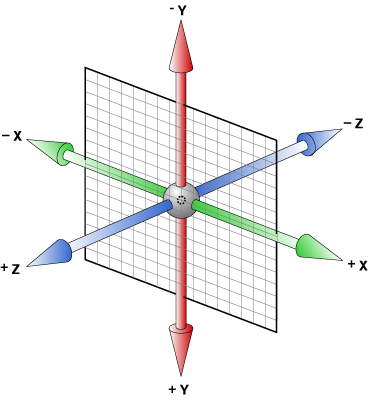# Rotate 3d## Watch out! vertical Y axis in 3d is inverted. Positive values are down

### We need to define an axis around which the element will turn.

The first point of the axis is the default transform-origin. We don't have to redefine it.
The second point of the axis is defined by giving the 3 first values for rotate3d property :

transform: rotate3d(x, y, z, rotation angle);

Rotate me!
```1 point: transform-origin by default

2 point : construct by
.rotated {
transform: rotate3d (1, -1, 0, 60deg);
}

```

i.e., x=1, y=-1, z=0, rotation angle = 60deg

Rotate me!
```1 point: transform-origin by default

2 point : construct by
.rotated {
transform: rotate3d (1, -1, 1, 60deg);
}

```

i.e., x=1, y=-1, z=1, rotation angle = 60deg